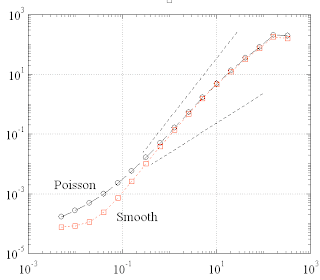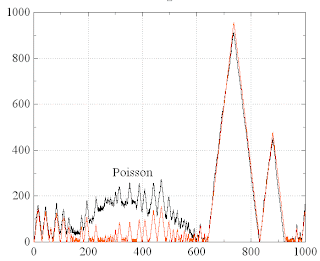## Wednesday, April 9, 2008

### [AD 9] "ZigZag" - Model of CSK remodelling

General concepts:

• We are interested in the spontaneous motion of CSK-bound micro-beads.
• In micro-particle rheology, it is common to explain the motion of the test particle by the interplay of two factors: First, there are external forces acting on the particle. Second, there is a rheological response function, which determines how the particle moves for a given external force profile.
• In our case of the cell, this distinction is not so obvious, since the bead is bound to an acto-myosin fiber network with active and passive components. The same network plays the role of a medium and also provides "external" forces, due to remodelling events.
• To make the conceptual problem clearer, let us imagine the fiber network as a mesh of linear springs with given spring constants and rest lengths. The bead is just a special node in this network. Its position is at any time at the mechanical equilibrium position.
• Since living biological systems are due to constant turnover and remodelling, the spring constants and rest lengths are temporally changing. In particular, stress fibers can contract (shrinking rest length) and thus create prestress in the network. Consequently, the beads equilibrium position is constantly shifted around. This resembles the observed spontaneous bead motion.
• Note that it is not clear in the above example how to separate the parts played by the medium and the forces. All we can say is that the parameters of our network are gradually changing and this is accompanied by shape changes of the network. It evolves through a succession of mechanical equilibrium configurations. After each remodelling "event", the prestress as well as the stiffness of certain fibers may have changed simultaneously.
• However, to simplify the analysis, we nevertheless stick to the medium/forces distinction. In particular, we will treat the forces in more detail than the medium in the following model.
• The properties of the medium can in principal be measured independently, namely by applying known forces (for example: a force ramp) to the test particle and observing the resulting motion. This must be done quickly enough to avoid remodelling during the test. Such experiments have been done and it was found that the CSK is predominantly elastic on such relatively small time scales.
• For further simplification, we describe the medium by a radially symmetric potential well (reasonable in the sense of a statistical average). The bead would rest in the center of the well, if the prestress forces of the fibers would not change with time.
• However, we should at least account for the correlated changes of stiffness and prestress in the remodelling network. As a reasonable approximation, one could set the radial stiffness coefficient of the elastic well at each moment to a value that is proportional to the momentary total prestress of the stress fiber network (sum over all individual fiber forces).
• In reality, the network may consist not only of active, contractile fibers, but might also contain a passive sub-network. The stiffness contribution of the passive sub-network might be independent from the remodelling of the active stress fibers. In the following model, we describe the passive contribution by a constant, minimum stiffness of the well, which remains even if all active fibers disappear. This passive constribution is however chosen much smaller than the average active constribution in the steady state.
• Besides the prestress forces of the fibers, there are also other, quite trivial reasons for random bead fluctuations in a real experiment, as for example noise in the detection system and thermal fluctuations. They can be described by white Gaussian noise of suitable variance, uncorrelated in the x- and y-directions. This positional noise is simply added to the simulated bead trajectories in the end.
• Assume for moment that the well stiffness is constant. Then the bead position is at any moment proportional to the sum of all prestress forces acting on the node=bead. A bead moving with constant velocity in the direction of a particular fiber means that the force of this fiber increases linearly with time.
• Since the MSD of the bead is a fractional powerlaw (at least above the noise floor), the fluctuations of the prestress of each fiber have to be long-time correlated, or must at least contain a very wide spectrum of short, medium, and very long lasting processes.
• For simplicity, we assume that the prestress of each fiber grows with a fixed average rate for a certain duration. Then it decreases with another rate until zero. A new triangular cycle starts immediately, however with a new random duration, drawn from a wide probablity distribution.
• Just as each cycle is statistially independent from the previous one, the triangular fluctuations of different fibers are also independent from each other. The fiber directions remain fixed in the model.
• The model as described so far can explain many features observed in the experiments, but not the positive kurtosis of the bead's step width distribution at small lag times. A positive kurtosis is obtained when we assume that changes of a fiber's prestress do not proceed continuously, but in a "quantized" manner, i.e. in discrete units.
• This is actually not unreasonable: A stress fiber can add a g-actin monomer to the end of a polymerizing filament. This increases the overlap between parallel filaments and offers new opportunities for myosin motors to crosslink. As a consequence, the prestress increases by a certain discrete amount (Note that the increase must not be prescisely the same each time).
• The adding or removing of structual, force-generating units to a stress fiber is a stochastic process. It can be most easily modelled by a Poisson process: The regulatory system of the cell prescribes only the average rates of the assembly/disassembly processes. The actual time of each single event is random with exponentially distributed inter-event times.
• The average assembly and disassembly rates are themselves changing, however in a more gradual manner. For simplicity, we assume that the disassembly rate is constant. The assembly rate is up-regulated to a value exceeding the disassembly rate during the growth phases of the fiber. It is down-regulated during the shrinking phases.

The model in detail (model parameters in red):
• NFib fibers are attached to the bead in radial spider geometry with statistically isotrope directions.
• The bead is bound in a radially symmetric elastic potential well with a prestress-dependent stiffness kWell(t).
• The fibers exert a temporaly changing prestresses F_n(t)=f_n(t)*unitvec_n onto the bead (reflecting growth and shrinking of the fibers by biochemical processes).
• The forces add up vectorially to F_sum(t).
• The bead's mechanical equilibrium position R(t) is at each moment given by F_sum(t)/kWell(t).
• Here, kWell(t) = kWell_Min + c * [sum_n f_n(t)].
• The fiber growth times forces are log-normal distributed: tGrow_n = 1sec * 10^GaussRnd(eMean,eFluct).
• Fibers grow with constant rate for a time tGrow_n. Then they decay with the same rate to zero force. Then another cycle starts with a new random tGrow_n.
• The above temporal changes of fiber force f(t) are generated by an imbalance between a constant background disassembly rate lamdaDis (unit: Newton/sec) and a varying assembly rate lambdaAss(t).
• In the model, lambdaAss(t) is switching between values 0 (shrinking phase) and lambdaAssMax (growing phase).
• The fibers grow/shrink with Poisson kinetics: Fibers change size in discrete units. Each additional unit increases the fiber force by an amount frcStp.
• A Gaussian white noise of variance sigma2Noise it added a posteriori to the bead position to simulate thermal and instrument noise.

Example of resulting MSD with and without (smooth) Poisson kinetics:And the kurtosis:The size of fiber 1 versus time:Simulation-Parameters:

NMX=10; // nr. of fibers
lambdaDis=10.0; // disassembly rate in [pN/sec]
lambdaAssMax=2.0*lambdaDis; // on value of assembly (fiber force increase) rate
eMean=0.0; // distribution of fiber growth times ,
eFluct=1.5; // tGrow_n = 1sec * 10^GaussRnd(eMean,eFluct)
frcStp=1.0; // force increase per assembled unit in [pN]
kStiff=100.0; // elastic stiffness in [pN/um]
sigma2Noise=1.0e-4; // white noise force amplitude (plateau height) in [um2/sec]